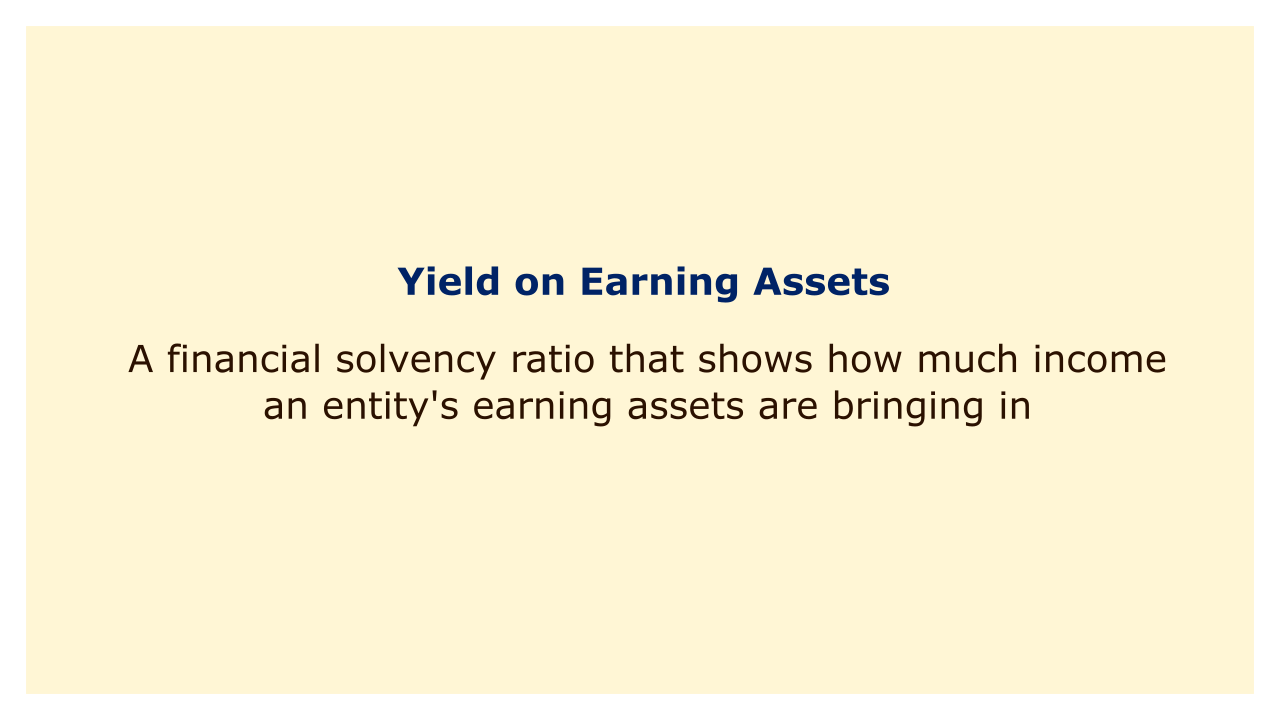# Yield on Earning AssetsImage: Moneybestpal.com

### The yield on earning assets is a measure of a company's financial soundness that reveals how much revenue its earning assets are producing.

Loans, securities, and investments are examples of earning assets since they produce interest income. Interest income is the revenue that a financial institution earns from lending money or investing in debt instruments.

The formula for calculating yield on earning assets is:

Yield on Earning Assets = Interest Income / Average Earning Assets

The average earning assets can be calculated by adding the beginning and ending balances of the earning assets and dividing by two. Alternatively, some analysts may use the total earning assets at the end of the period.

#### Why Is Yield on Earning Assets Important?

Yield on earning assets is important because it indicates how well a financial institution is using its assets to generate income. A high yield on earning assets indicates that, in relation to the value of those assets, the organization is receiving a significant amount of interest income. This suggests that the company is making effective and efficient use of its resources and that it enjoys a competitive advantage in the market.

A low yield on earning assets indicates that, in relation to the value of such assets, the organization is only receiving a modest amount of interest revenue. This suggests that the company is employing its resources inefficiently and poorly, which puts it at a competitive disadvantage in the market.

A low yield on earning assets also suggests that the organization might have trouble satisfying its immediate obligations and might be in danger of defaulting or going bankrupt. This is the reason why when assessing the stability and performance of a financial institution, regulators and investors pay particular attention to this ratio.

#### How to Improve Yield on Earning Assets?

There are several ways that a financial institution can improve its yield on earning assets, such as:
• Raising the rates of interest for loans and investments
• Lowering the cost of financing and obligations
• Including higher-yielding, lower-risk investments in the portfolio of earning assets
• Enhancing the effectiveness and quality of loans and investments
• Expanding both the customer base and the market share
• Better pricing and risk management practices

However, improving yield on earning assets also involves trade-offs and challenges, such as:
• Weighing the risk and reward on investment in assets
• Maintaining sufficient capital and liquidity ratios
• Adhering to legal criteria and norms
• Battling competition and changing market conditions
• Controlling client happiness and expectations

In order to optimize its profitability and solvency without jeopardizing its stability and reputation, a financial institution should strive for the optimal amount of yield on earning assets.

#### Example of Yield on Earning Assets

Let's take a look at a hypothetical bank's illustration of how to compute and interpret yield on earning assets. The bank reported the following information for the year 2022:
• Interest income: \$10 million
• Beginning earning assets: \$100 million
• Ending earning assets: \$120 million

Using the formula above, we can calculate the yield on earning assets as follows:

Yield on Earning Assets = Interest Income / Average Earning Assets

= \$10 million / [(\$100 million + \$120 million) / 2]

= \$10 million / \$110 million

= 0.0909 or 9.09%

This means that for every dollar of earning assets, the bank earned 9.09 cents of interest income in 2022.

We can compare this ratio to the average for the sector or to banks that are comparable to them in order to evaluate it. In 2022, the bank would have performed better than average if, for instance, the industry average yield on earning assets was 8%. In contrast, if an other bank's yield on earning assets was 10%, we could conclude that in 2022, the other bank had outperformed it.
Tags